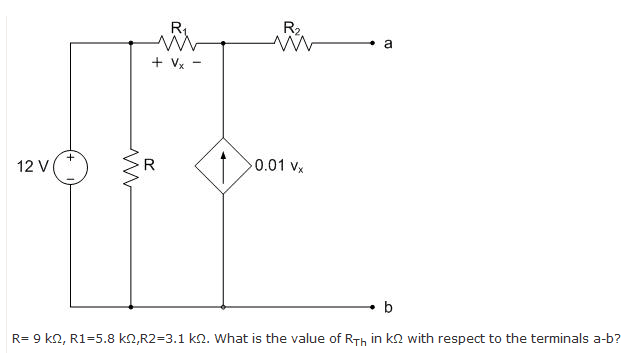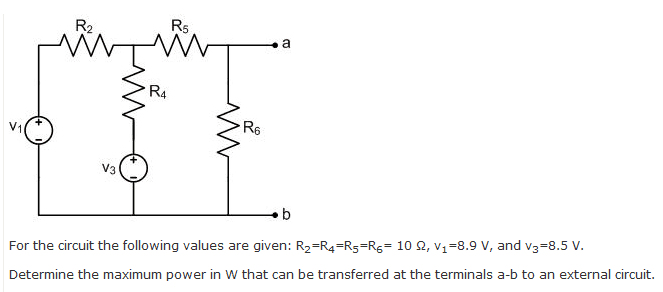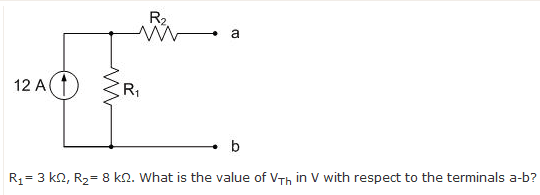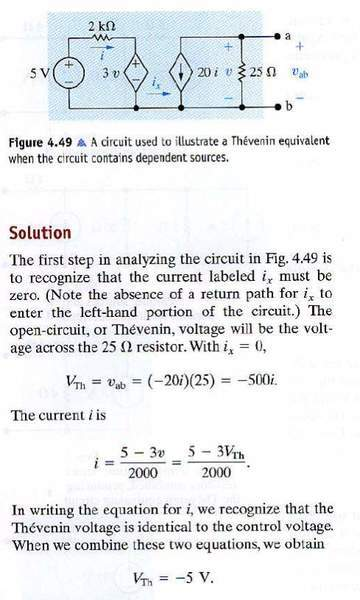# Electric Circuit. Norton Equivalent current

Hitman6267
Electric Circuit. Norton/Thevelin Equivalents

Unsolved ProblemI used a node voltage equation to try and get Vth and the I in the short-circuit to get the Rth but it didn't work.

Unsolved ProblemI used a node voltage equation to get Vth and I in the short-circuit. Got Rth and used in the in Vth^2 / 4 RL (with RL = Rth)

My answer was wrong. Is the method correct ?

Solved this my self
Removed to make space for another problem

For me, I find that the Norton equivalent is going to be 12A since the independent current source forces that ampage. But apparently I'm wrong. (I get feedback from a web form when I submit the answer). Any one can tell me whats wrong with my thought process ? Thank you.

Solution:
After doing a source transformation and adding up the resistors. You can get the correct the Norton current value.

SolvedI thought that if I did a source transformation getting a 36000V source would solve the problem because there isn't any other voltage source in the circuit. I was wrong. Any hints on this one ?

Last edited:

Staff Emeritus
Homework Helper

Unsolved Problem
View attachment 24900

I thought that if I did a source transformation getting a 36000V source would solve the problem because there isn't any other voltage source in the circuit. I was wrong. Any hints on this one ?
You're right. The Thevenin voltage is the open-circuit voltage, which is 36000 V.

Hitman6267
Yes it turns out my answer is correct and that they forgot to ask for the answer in kilo ohm.Can any one tell me why the the Vth is equal to the v in the dependent source "3V"

A solution says i = $$\frac{5-3V}{2000}$$ = $$\frac{5-3Vth}{2000}$$

Last edited:
Staff Emeritus
Homework Helper